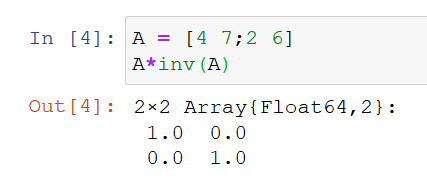Open In App

# Manipulating matrices in Julia

Matrices in Julia are the heterogeneous type of containers and hence, they can hold elements of any data type. It is not mandatory to define the data type of a matrix before assigning the elements to the matrix. Julia automatically decides the data type of the matrix by analyzing the values assigned to it. Because of the ordered nature of a matrix, it makes it easier to perform operations on its values based on their index.

Following are some common matrix manipulation operations in Julia:

• Transpose of a matrix
• Flipping a matrix
• Concatenating matrices
• Reshaping a matrix
• Inverse of a matrix

#### Creating a matrix

Julia provides a very simple notation to create matrices. A matrix can be created using the following notation: A = [1 2 3; 4 5 6]. Spaces separate entries in a row and semicolons separate rows. We can also get the size of a matrix using size(A).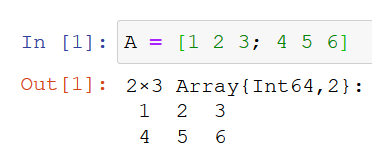#### Transpose of a matrix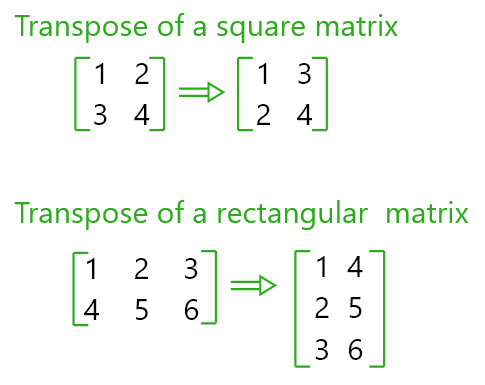• The transpose operation flips the matrix over its diagonal by switching the rows and columns.
• Let A be a matrix. We can get the transpose of A by using A’.
• Example 1:

 `# Defining a square matrix of size (2, 2)``A ``=` `[``1` `2``; ``3` `4``]    `` ` `# Transpose of A ``A'               `

Output: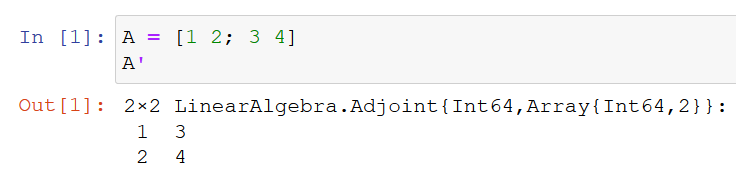Example 2:

 `# Defining a retangular matrix of size (2, 3)``B ``=` `[``1` `2` `3``; ``4` `5` `6``]   `` ` `# Transpose of B``B'                   `

Output: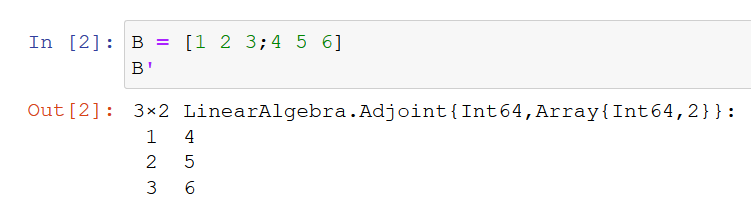#### Flipping a matrix: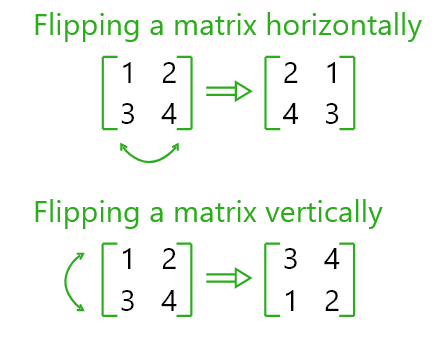• A matrix in Julia can be flipped via the X-axis i.e. horizontally or via the Y-axis i.e. vertically.
• To flip the matrix we use `reverse(< matrix >, dims= < 1 or 2 >))` 1 = vertically, 2 = horizontally.

Example 1: Flipping vertically

 `# Defining a rectangular matrix of size (2, 3)``B ``=` `[``1` `2` `3``; ``4` `5` `6``]    `` ` `# Flipping the matrix vertically``reverse(B, dims ``=` `1``)   `

Output: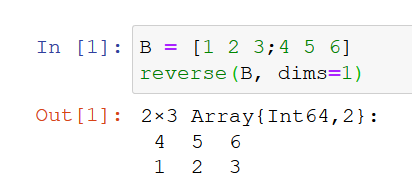Example 2: Flipping horizontally

 `# Flipping the matrix horizontally``reverse(B, dims ``=` `2``)   `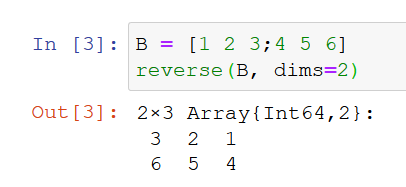#### Concatenating matrices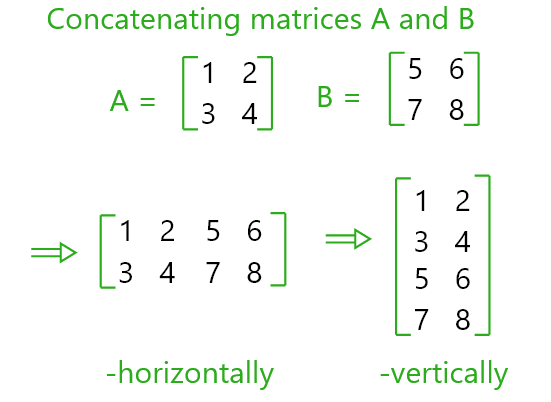• In Julia we can concatenate a matrix to another matrix to the right side of the initial matrix or to the bottom of it.
• We use `vcat(A, B)` to concatenate to the side.
• And `hcat(A, B)` to concatenate to the bottom.
• While concatenating to the side, we need to make sure that both the matrices have same number of rows.
• While concatenating to the bottom, we need to make sure that both the matrices have same number of columns.

Example 1: Concatenate to the side

 `# Creating a square matrix of size (2, 2)``A ``=` `[``1` `2``; ``3` `4``]        `` ` `# Creating a rectangular matrix of size (2, 3)``B ``=` `[``5` `6` `7``; ``8` `9` `10``]   ``hcat(A, B)`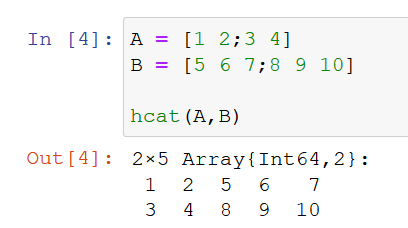Example 2: Concatenate to the bottom

 `# Creating a square matrix of size (3, 2)``A ``=` `[``1` `2``;``3` `4``; ``5` `6``]        `` ` `# Creating a rectangular matrix of size (4, 2)``B ``=` `[``5` `7``;``8` `9``; ``10` `11``;``14` `16``]   ``vcat(A, B)`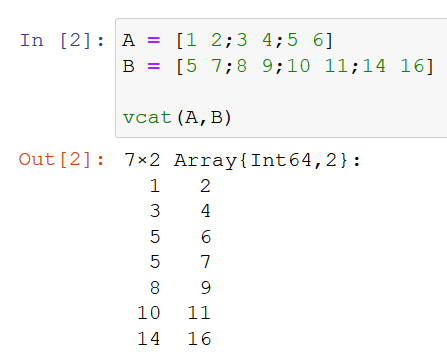#### Reshaping a matrix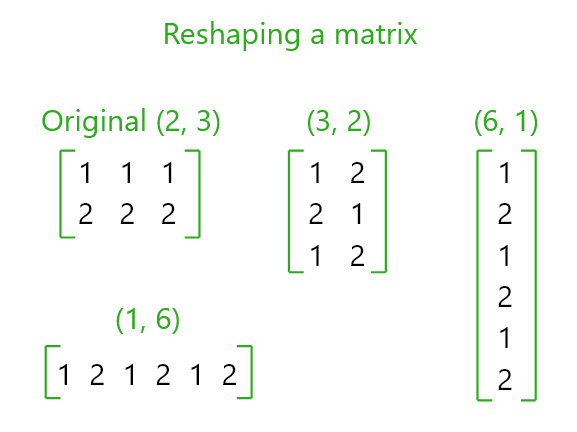We can reshape a matrix into another matrix of different size.
Example 1: Reshaping a matrix

 `# The original matrix with size (3, 2)``A ``=` `[``1` `2``; ``3` `4``; ``5` `6``]    `

• Output:
•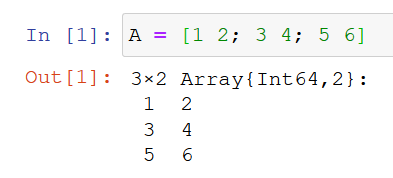Reshaping the matrix to size (2, 3)

 `reshape(A, (``2``, ``3``))`

Output: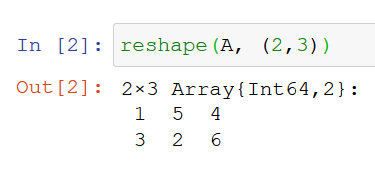Reshaping the matrix to size (6, 1)

 `reshape(A, (``6``, ``1``))`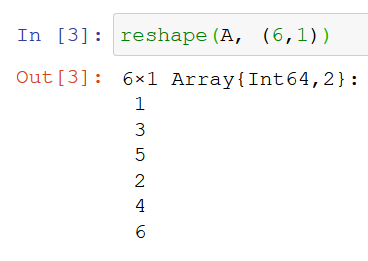Reshaping the matrix to size (1, 6)

 `reshape(A, (``1``, ``6``))`

Output: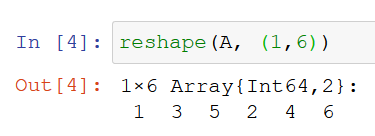#### Inverse of a matrix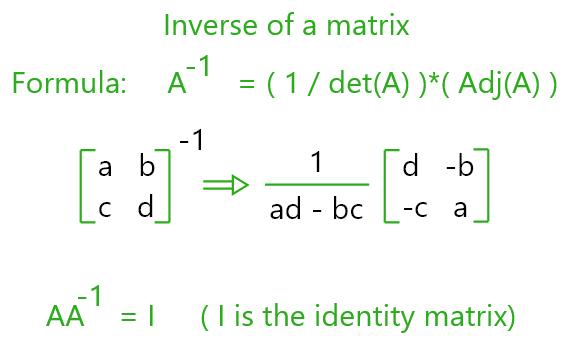• If A is a square matrix its multiplicative inverse is called its inverse matrix. Denoted by A-1.
• In Julia we use `inv(A)` to get the inverse of the matrix A.
• Example 1: Getting the Inverse of a matrix

 `# Creating a square matrix of size (2, 2)``A ``=` `[``4` `7``; ``2` `6``]      `` ` `# Getting the inverse of matrix A  ``inv(A)               `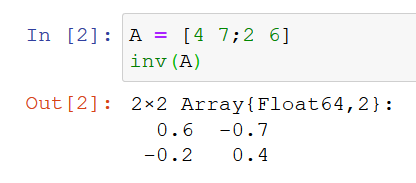Example 2: Getting Identity Matrix

 `# Creating a square matrix of size (2, 2)``A ``=` `[``4` `7``; ``2` `6``]     `` ` `# Getting the Identity matrix``A ``*` `inv(A)         `